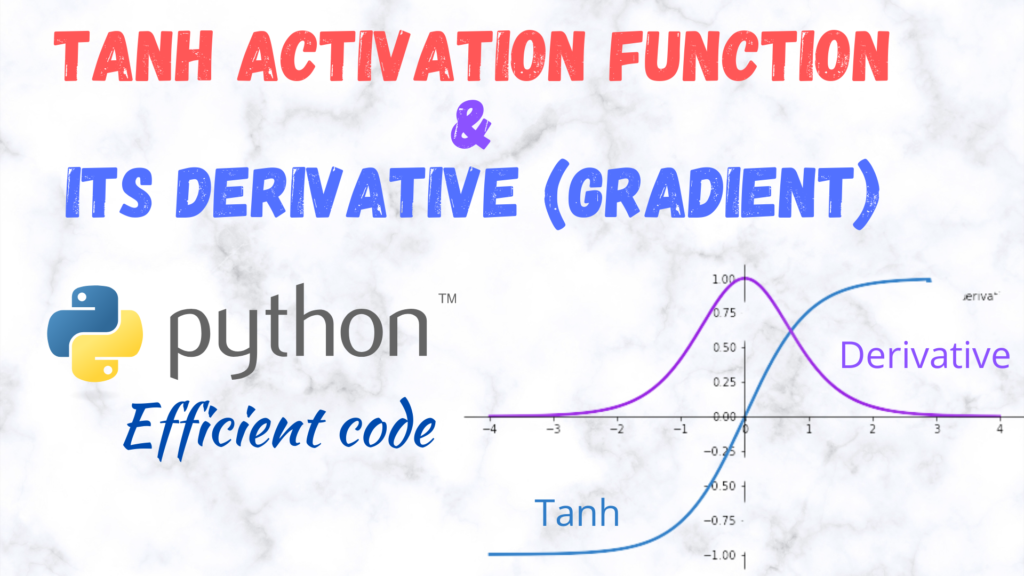# Efficient implementation of Tanh activation function and its Derivative (gradient) in PythonThe mathematical definition of the Tanh activation function is$f(x)= \tanh(x)$

and its derivative is defined as$f'(x)= 1-\tanh ^2(x)$

The Tanh function and its derivative for a batch of inputs (a 2D array with nRows=nSamples and nColumns=nNodes) can be implemented in the following manner:
Tanh simplest implementation

```import numpy as np
def Tanh(x):
return np.tanh(x)
```

Tanh derivative simplest implementation

```import numpy as np
return 1.-np.tanh(x)**2 # sech^2{x}
```

However, these implementations can be further accelerated (sped-up) by using Numba (https://numba.pydata.org/). Numba is a Just-in-time (JIT) compiler that

translates a subset of Python and NumPy code into fast machine code.

To use numba, install it as:

```pip install numba
```

Also, make sure that your numpy is compatible with Numba or not, although usually pip takes care of that. You can get the info here: https://pypi.org/project/numba/

Accelerating the above functions using Numba is quite simple. Just modify them in the following manner:

Tanh NUMBA implementation

```from numba import njit
@njit(cache=True,fastmath=True)
def Tanh(x):
return np.tanh(x)
```

Tanh derivative NUMBA implementation

```from numba import njit
@njit(cache=True,fastmath=True)
return 1.-np.tanh(x)**2 # sech^2{x}
```

This is quite fast and competitive with Tensorflow and PyTorch (https://github.com/manassharma07/crysx_nn/blob/main/benchmarks_tests/Performance_Activation_Functions_CPU.ipynb).

It is in fact also used in the CrysX-Neural Network library (crysx_nn)

Furthermore, the above implementations can be further accelerated using Cupy (CUDA), if using single precision (float32) is not a problem.

CuPy is an open-source array library for GPU-accelerated computing with Python. CuPy utilizes CUDA Toolkit libraries to make full use of the GPU architecture.

The Cupy implementations look as follows:

```import cupy as cp
def Tanh_cupy(x):
return cp.tanh(x)
```
```def Tanh_grad_cupy(x):
return 1.-cp.tanh(x)**2 # sech^2{x}
```

The above code is also used in the crysx_nn library.
To see how the crysx_nn implementations of Tanh compare with TensorFlow and PyTorch, click here.

I hope you found this information useful.

If you did, then don’t forget to check out my other posts on Machine Learning and efficient implementations of activation/loss functions in Python.[wpedon id="7041" align="center"]